# Platonic solids

A Platonic solid is a regular solid in which every face is the same regular polygon and all the sides meet at the same angles at each vertex and all the faces meet at the same angles at each edge.

In the list below the number of faces, edges and vertices are listed as (F, E, V).

#### F, E, V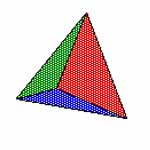Tetrahedron

4 triangles
4, 6, 4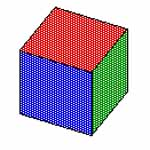Cube

6 squares
6, 12, 8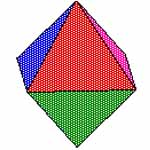Octahedron

8 triangles
8, 12, 6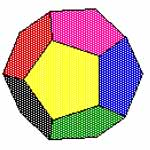Dodecahedron

12 pentagons
12, 30, 20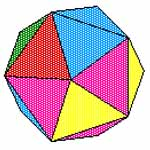Icosahedron

20 triangles
20, 30, 12